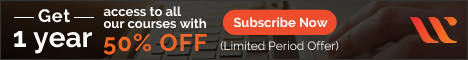# 7 Numpy Practical Examples: Sample Code for BeginnersIn the previous tutorial, we have discussed some basic concepts of NumPy in Python Numpy Tutorial For Beginners With Examples. In this tutorial, we are going to discuss some problems and the solution with NumPy practical examples and code.

As you might know, NumPy is one of the important Python modules used in the field of data science and machine learning. As a beginner, it is very important to know about a few NumPy practical examples.

## Numpy Practical Examples

Let’s have a look at 7 NumPy sample solutions covering some key NumPy concepts. Each example has code with a relevant NumPy library and its output.

### How to search the maximum and minimum element in the given array using NumPy?

Searching is a technique that helps finds the place of a given element or value in the list. In Numpy, one can perform various searching operations using the various functions that are provided in the library like argmax, argmin, etc.

1. numpy.argmax( )

This function returns indices of the maximum…